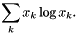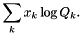# utilities.h File Reference

Various templated functions that carry out common vector operations (see Global Utility Functions). More...

#include "ct_defs.h"
Include dependency graph for utilities.h:This graph shows which files directly or indirectly include this file:Go to the source code of this file.

## Classes

struct  timesConstant< T >
Unary operator to multiply the argument by a constant. More...

## Namespaces

namespace  Cantera

Namespace for the Cantera kernel.

## Functions

template<class T , class S >
max (T i, S j)
Maximum of two templated quantities, i and j.
template<class T , class S >
min (T i, S j)
Minimum of two templated quantities, i and j.
template<class V >
doublereal dot4 (const V &x, const V &y)
Templated Inner product of two vectors of length 4.
template<class V >
doublereal dot5 (const V &x, const V &y)
Tempalted Inner product of two vectors of length 5.
template<class V >
doublereal dot6 (const V &x, const V &y)
Tempalted Inner product of two vectors of length 6.
template<class InputIter , class InputIter2 >
doublereal dot (InputIter x_begin, InputIter x_end, InputIter2 y_begin)
Function that calculates a templated inner product.
template<class InputIter , class OutputIter , class S >
void scale (InputIter begin, InputIter end, OutputIter out, S scale_factor)
Multiply elements of an array by a scale factor.
template<class InputIter , class OutputIter , class S >
void increment_scale (InputIter begin, InputIter end, OutputIter out, S scale_factor)
template<class InputIter , class OutputIter >
void multiply_each (OutputIter x_begin, OutputIter x_end, InputIter y_begin)
Multiply each entry in x by the corresponding entry in y.
template<class InputIter >
void resize_each (int m, InputIter begin, InputIter end)
Invoke method 'resize' with argument m for a sequence of objects (templated version).
template<class InputIter >
doublereal absmax (InputIter begin, InputIter end)
The maximum absolute value (templated version).
template<class InputIter , class OutputIter >
void normalize (InputIter begin, InputIter end, OutputIter out)
Normalize the values in a sequence, such that they sum to 1.0 (templated version).
template<class InputIter , class OutputIter >
void divide_each (OutputIter x_begin, OutputIter x_end, InputIter y_begin)
Templated divide of each element of x by the corresponding element of y.
template<class InputIter , class OutputIter >
void sum_each (OutputIter x_begin, OutputIter x_end, InputIter y_begin)
Increment each entry in x by the corresponding entry in y.
template<class InputIter , class OutputIter , class IndexIter >
void scatter_copy (InputIter begin, InputIter end, OutputIter result, IndexIter index)
Copies a contiguous range in a sequence to indexed positions in another sequence.
template<class InputIter , class RandAccessIter , class IndexIter >
void scatter_mult (InputIter mult_begin, InputIter mult_end, RandAccessIter data, IndexIter index)
Multiply selected elements in an array by a contiguous sequence of multipliers.
template<class InputIter , class OutputIter , class IndexIter >
void scatter_divide (InputIter begin, InputIter end, OutputIter result, IndexIter index)
Divide selected elements in an array by a contiguous sequence of divisors.
template<class InputIter >
doublereal sum_xlogx (InputIter begin, InputIter end)
Compute.

template<class InputIter1 , class InputIter2 >
doublereal sum_xlogQ (InputIter1 begin, InputIter1 end, InputIter2 Q_begin)
Compute.

template<class OutputIter >
void scale (int N, double alpha, OutputIter x)
Scale a templated vector by a constant factor.
template<class D , class R >
poly6 (D x, R *c)
Templated evaluation of a polynomial of order 6.
template<class D , class R >
poly8 (D x, R *c)
Templated evaluation of a polynomial of order 8.
template<class D , class R >
poly10 (D x, R *c)
Templated evaluation of a polynomial of order 10.
template<class D , class R >
poly5 (D x, R *c)
Templated evaluation of a polynomial of order 5.
template<class D , class R >
poly4 (D x, R *c)
Evaluates a polynomial of order 4.
template<class D , class R >
poly3 (D x, R *c)
Templated evaluation of a polynomial of order 3.

## Detailed Description

Various templated functions that carry out common vector operations (see Global Utility Functions).

Definition in file utilities.h.

Generated by1.6.3